Mathematics Commons™

17,858 Full-Text Articles 17,112 Authors 4,137,002 Downloads285 Institutions

All Articles in Mathematics

17,858 full-text articles. Page 5 of 528.

Cyclotomic Trace Codes, 2019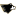University of Rijeka

Cyclotomic Trace Codes, Dean Crnkovic, Andrea Svob, Vladimir Tonchev

Michigan Tech Publications

A generalization of Ding’s construction is proposed that employs as a defining set the collection of the sth powers (s ≥ 2) of all nonzero elements in GF(pm), where p ≥ 2 is prime. Some of the resulting codes are optimal or near-optimal and include projective codes over GF(4) that give rise to optimal or near optimal quantum codes. In addition, the codes yield interesting combinatorial structures, such as strongly regular graphs and block designs.

2019University of Florence

Generalization Of Real Interval Matrices To Other Fields, Elena Rubei

An interval matrix is a matrix whose entries are intervals in $\R$. This concept, which has been broadly studied, is generalized to other fields. Precisely, a rational interval matrix is defined to be a matrix whose entries are intervals in $\Q$. It is proved that a (real) interval $p \times q$ matrix with the endpoints of all its entries in $\Q$ contains a rank-one matrix if and only if it contains a rational rank-one matrix, and contains a matrix with rank smaller than $\min\{p,q\}$ if and only if it contains a rational matrix with rank smaller than $\min ... Analysis Of Feast Spectral Approximations Using The Dpg Discretization, 2019Portland State University Analysis Of Feast Spectral Approximations Using The Dpg Discretization, Jay Gopalakrishnan, Luka Grubišić, Jeffrey S. Ovall, Benjamin Q. Parker Jeffrey S. Ovall A filtered subspace iteration for computing a cluster of eigenvalues and its accompanying eigenspace, known as “FEAST”, has gained considerable attention in recent years. This work studies issues that arise when FEAST is applied to compute part of the spectrum of an unbounded partial differential operator. Specifically, when the resolvent of the partial differential operator is approximated by the discontinuous Petrov Galerkin (DPG) method, it is shown that there is no spectral pollution. The theory also provides bounds on the discretization errors in the spectral approximations. Numerical experiments for simple operators illustrate the theory and also indicate the value of ... Torsors Over Simplicial Schemes, 2019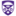The University of Western Ontario Torsors Over Simplicial Schemes, Alexander S. Rolle Electronic Thesis and Dissertation Repository Let X be a simplicial object in a small Grothendieck site C, and let G be a sheaf of groups on C. We define a notion of G-torsor over X, generalizing a definition of Gillet, and prove that there is a bijection between the set of isomorphism classes of G-torsors over X, and the set of maps in the homotopy category of simplicial presheaves on C, with respect to the local weak equivalences, from X to BG. We prove basic results about the resulting non-abelian cohomology invariant, including an exact sequence associated to a central extension of sheaves of groups ... 2019The University of Western Ontario Polynomial And Rational Convexity Of Submanifolds Of Euclidean Complex Space, Octavian Mitrea Electronic Thesis and Dissertation Repository The goal of this dissertation is to prove two results which are essentially independent, but which do connect to each other via their direct applications to approximation theory, symplectic geometry, topology and Banach algebras. First we show that every smooth totally real compact surface in complex Euclidean space of dimension 2 with finitely many isolated singular points of the open Whitney umbrella type is locally polynomially convex. The second result is a characterization of the rational convexity of a general class of totally real compact immersions in complex Euclidean space of dimension n.. Analysis Of Feast Spectral Approximations Using The Dpg Discretization, 2019Portland State University Analysis Of Feast Spectral Approximations Using The Dpg Discretization, Jay Gopalakrishnan, Luka Grubišić, Jeffrey S. Ovall, Benjamin Q. Parker Jay Gopalakrishnan A filtered subspace iteration for computing a cluster of eigenvalues and its accompanying eigenspace, known as “FEAST”, has gained considerable attention in recent years. This work studies issues that arise when FEAST is applied to compute part of the spectrum of an unbounded partial differential operator. Specifically, when the resolvent of the partial differential operator is approximated by the discontinuous Petrov Galerkin (DPG) method, it is shown that there is no spectral pollution. The theory also provides bounds on the discretization errors in the spectral approximations. Numerical experiments for simple operators illustrate the theory and also indicate the value of ... The Dpg-Star Method, 2019University of Texas at Austin The Dpg-Star Method, Leszek Demkowicz, Jay Gopalakrishnan, Brendan Keith Jay Gopalakrishnan This article introduces the DPG-star (from now on, denoted DPG*) finite element method. It is a method that is in some sense dual to the discontinuous Petrov– Galerkin (DPG) method. The DPG methodology can be viewed as a means to solve an overdetermined discretization of a boundary value problem. In the same vein, the DPG* methodology is a means to solve an underdetermined discretization. These two viewpoints are developed by embedding the same operator equation into two different saddle-point problems. The analyses of the two problems have many common elements. Comparison to othermethods in the literature round out the newly ... Classification Of Minimal Separating Sets In Low Genus Surfaces, 2019Portland State University Classification Of Minimal Separating Sets In Low Genus Surfaces, J. J. P. Veerman, William Maxwell, Victor Rielly, Austin K. Williams J. J. P. Veerman Consider a surface S and let MS. If S \ M is not connected, then we say M separates S, and we refer to M as a separating set of S. If M separates S, and no proper subset of M separates S, then we say M is a minimal separating set of S. In this paper we use computational methods of combinatorial topology to classify the minimal separating sets of the orientable surfaces of genus g = 2 and g = 3. The classification for genus 0 and 1 was done in earlier work, using methods of algebraic topology. 2019The University of Western Ontario Of Matroid Polytopes, Chow Rings And Character Polynomials, Ahmed Ashraf Electronic Thesis and Dissertation Repository Matroids are combinatorial structures that capture various notions of independence. Recently there has been great interest in studying various matroid invariants. In this thesis, we study two such invariants: Volume of matroid base polytopes and the Tutte polynomial. We gave an approach to computing volume of matroid base polytopes using cyclic flats and apply it to the case of sparse paving matroids. For the Tutte polynomial, we recover (some of) its coefficients as degrees of certain forms in the Chow ring of underlying matroid. Lastly, we study the stability of characters of the symmetric group via character polynomials. We show ... Essential Dimension Of Parabolic Bundles, 2019The University of Western Ontario Essential Dimension Of Parabolic Bundles, Dinesh Valluri Electronic Thesis and Dissertation Repository Essential dimension of a geometric object is roughly the number of algebraically independent parameters needed to define the object. In this thesis we give upper bounds for the essential dimension of parabolic bundles over a non-singular curve X of genus g greater than or equal to 2 using Borne's correspondence between parabolic bundles on a curve and vector bundles on a root stack. This is a generalization of the work of Biswas, Dhillon and Hoffmann on the essential dimension of vector bundles, by following their method for curves and adapting it to root stacks. In this process, we invoke ... 2019The University of Western Ontario Ricci Curvature Of Noncommutative Three Tori, Entropy, And Second Quantization, Rui Dong Electronic Thesis and Dissertation Repository In noncommutative geometry, the metric information of a noncommutative space is encoded in the data of a spectral triple$(\mathcal{A}, \mathcal{H},D)$, where$D$plays the role of the Dirac operator acting on the Hilbert space of spinors. Ideas of spectral geometry can then be used to define suitable notions such as volume, scalar curvature, and Ricci curvature. In particular, one can construct the Ricci curvature from the asymptotic expansion of the heat trace$\textrm{Tr}(e^{-tD^2})\$. In Chapter 2, we will compute the Ricci curvature of a curved noncommutative three torus. The computation is done ...

Leveraging Variation Of Historical Number Systems To Build Understanding Of The Base-Ten Place-Value System, 2019Portland State University

Leveraging Variation Of Historical Number Systems To Build Understanding Of The Base-Ten Place-Value System, Eva Thanheiser, Kathleen Melhuish

Eva Thanheiser

Prospective elementary school teachers (PTs) come to their mathematics courses fluent in using procedures for adding and subtracting multidigit whole numbers, but many are unaware of the essential features inherent in understanding the base-ten place-value system (i.e., grouping, place value, base). Understanding these features is crucial to understanding and teaching number and place value. The research aims of this paper are (1) to present a local instructional theory (LIT), designed to familiarize PTs with these features through comparison with historical number systems and (2) to present the effects of using the LIT in the PT classroom. A theory of ...

General Nonlinear-Material Elasticity In Classical One-Dimensional Solid Mechanics, 2019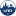University of New Orleans

General Nonlinear-Material Elasticity In Classical One-Dimensional Solid Mechanics, Ronald Joseph Giardina Jr

University of New Orleans Theses and Dissertations

We will create a class of generalized ellipses and explore their ability to define a distance on a space and generate continuous, periodic functions. Connections between these continuous, periodic functions and the generalizations of trigonometric functions known in the literature shall be established along with connections between these generalized ellipses and some spectrahedral projections onto the plane, more specifically the well-known multifocal ellipses. The superellipse, or Lam\'{e} curve, will be a special case of the generalized ellipse. Applications of these generalized ellipses shall be explored with regards to some one-dimensional systems of classical mechanics. We will adopt the Ramberg-Osgood ...

Mathamigos: A Community Mathematics Initiative, 2019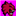Math Circles Collaborative of New Mexico

Mathamigos: A Community Mathematics Initiative, James C. Taylor, Delara Sharma, Shannon Rogers

Journal of Math Circles

We present a broad, and we think novel, community mathematics initiative in its early stages in Santa Fe, New Mexico. At every level, the program embraces community-wide collaboration—from the leadership team, to the elements of the mathematics being implemented (primarily math circles and the Global Math Project’s Exploding Dots), to the funding model. Our MathAmigos program falls within two categories of math circle-related programs: outreach and professional development (PD). In outreach, we work with the Santa Fe Public School district (administration, teachers, students, and parents) and the City of Santa Fe government (our funders via a two-year contract ...

Connecting Mathematics And Community: Challenges, Successes, And Different Perspectives, 2019Brown University

Connecting Mathematics And Community: Challenges, Successes, And Different Perspectives, Ariel Azbel, Margarita Azbel, Isabella F. Delbakhsh, Tami E. Heletz, Zeynep Teymuroglu

Journal of Math Circles

In this article, we summarize our personal journey to establish a successful math circle in a community that is not very familiar with such mathematics enrichment programs. We share the story of how our math circle began three years ago, as well as the lessons we learned and our organizational challenges and successes. Additionally, we outline three primary perspectives: the founder perspective, the student volunteer perspective, and the faculty volunteer perspective.

The Derived Category Of A Locally Complete Intersection Ring, 2019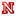University of Nebraska - Lincoln

The Derived Category Of A Locally Complete Intersection Ring, Joshua Pollitz

Dissertations, Theses, and Student Research Papers in Mathematics

Let R be a commutative noetherian ring. A well-known theorem in commutative algebra states that R is regular if and only if every complex with finitely generated homology is a perfect complex. This homological and derived category characterization of a regular ring yields important ring theoretic information; for example, this characterization solved the well-known localization problem" for regular local rings. The main result of this thesis is establishing an analogous characterization for when R is locally a complete intersection. Namely, R is locally a complete intersection if and only if each nontrivial complex with finitely generated homology can build a ...

The T3,T4-Conjecture For Links, 2019University of Nebraska - Lincoln

The T3,T4-Conjecture For Links, Katie Tucker

Dissertations, Theses, and Student Research Papers in Mathematics

An oriented n-component link is a smooth embedding of n oriented copies of S1 into S3. A diagram of an oriented link is a projection of a link onto R2 such that there are no triple intersections, with notation at double intersections to indicate under and over strands and arrows on strands to indicate orientation. A local move on an oriented link is a regional change of a diagram where one tangle is replaced with another in a way that preserves orientation. We investigate the local moves t3 and t4, which are conjectured to ...

Introduction To Classical Field Theory, 2019Department of Physics, Utah State University

Introduction To Classical Field Theory, Charles G. Torre

All Complete Monographs

This is an introduction to classical field theory. Topics treated include: Klein-Gordon field, electromagnetic field, scalar electrodynamics, Dirac field, Yang-Mills field, gravitational field, Noether theorems relating symmetries and conservation laws, spontaneous symmetry breaking, Lagrangian and Hamiltonian formalisms.

2019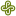Portland State University

Discretization Of The Hellinger-Reissner Variational Form Of Linear Elasticity Equations, Kevin A. Sweet

REU Final Reports

This paper addresses the derivation of the Hellinger-Reissner Variational Form from the strong form of a system of linear elasticity equations that are used in relation to geological phenomena. The problem is discretized using finite element discretization. This allowed the creation of a program that was used to run tests on various domains. The resultant displacement vectors for tested domains are shown at the end of the paper.

Machine Learning Techniques As Applied To Discrete And Combinatorial Structures, 2019Utah State University

Machine Learning Techniques As Applied To Discrete And Combinatorial Structures, Samuel David Schwartz

All Graduate Theses and Dissertations

Machine Learning Techniques have been used on a wide array of input types: images, sound waves, text, and so forth. In articulating these input types to the almighty machine, there have been all sorts of amazing problems that have been solved for many practical purposes.

Nevertheless, there are some input types which don’t lend themselves nicely to the standard set of machine learning tools we have. Moreover, there are some provably difficult problems which are abysmally hard to solve within a reasonable time frame.

This thesis addresses several of these difficult problems. It frames these problems such that we ...